> > Hack the virtual memory: the stack, registers and assembly code

# Hack the virtual memory: the stack, registers and assembly codeThis is the fifth chapter in a series about virtual memory. The goal is to learn some CS basics in a different and more practical way.

If you missed the previous chapters, you should probably start there:

## The Stack

As we have seen in chapter 2, the stack resides at the high end of memory and grows downward. But how does it work exactly? How does it translate into assembly code? What are the registers used? In this chapter we will have a closer look at how the stack works, and how the program automatically allocates and de-allocates local variables.

Once we understand this, we will be able to play a bit with it, and hijack the flow of our program. Ready? Let’s start!

Note: We will talk only about the user stack, as opposed to the kernel stack

## Prerequisites

In order to fully understand this article, you will need to know:

• The basics of the C programming language (especially pointers)

## Environment

All scripts and programs have been tested on the following system:

• Ubuntu
• Linux ubuntu 4.4.0-31-generic #50~14.04.1-Ubuntu SMP Wed Jul 13 01:07:32 UTC 2016 x86_64 x86_64 x86_64 GNU/Linux
• Tools used:
• gcc
• gcc (Ubuntu 4.8.4-2ubuntu1~14.04.3) 4.8.4
• objdump
• GNU objdump (GNU Binutils for Ubuntu) 2.2

Everything we cover will be true for this system/environment, but may be different on another system

## Automatic allocation

Let’s first look at a very simple program that has one function that uses one variable (`0-main.c`):

``````#include <stdio.h>

int main(void)
{
int a;

a = 972;
printf("a = %d\n", a);
return (0);
}
``````

Let’s compile this program and disassemble it using `objdump`:

``````holberton\$ gcc 0-main.c
holberton\$ objdump -d -j .text -M intel
``````

The assembly code produced for our `main` function is the following:

``````000000000040052d <main>:
40052d:       55                      push   rbp
40052e:       48 89 e5                mov    rbp,rsp
400531:       48 83 ec 10             sub    rsp,0x10
400535:       c7 45 fc cc 03 00 00    mov    DWORD PTR [rbp-0x4],0x3cc
40053c:       8b 45 fc                mov    eax,DWORD PTR [rbp-0x4]
40053f:       89 c6                   mov    esi,eax
400541:       bf e4 05 40 00          mov    edi,0x4005e4
400546:       b8 00 00 00 00          mov    eax,0x0
40054b:       e8 c0 fe ff ff          call   400410 <printf@plt>
400550:       b8 00 00 00 00          mov    eax,0x0
400555:       c9                      leave
400556:       c3                      ret
400557:       66 0f 1f 84 00 00 00    nop    WORD PTR [rax+rax*1+0x0]
40055e:       00 00
``````

Let’s focus on the first three lines for now:

``````000000000040052d <main>:
40052d:       55                      push   rbp
40052e:       48 89 e5                mov    rbp,rsp
400531:       48 83 ec 10             sub    rsp,0x10
``````

The first lines of the function `main` refers to `rbp` and `rsp`; these are special purpose registers. `rbp` is the base pointer, which points to the base of the current stack frame, and `rsp` is the stack pointer, which points to the top of the current stack frame.

Let’s decompose step by step what is happening here. This is the state of the stack when we enter the function `main` before the first instruction is run: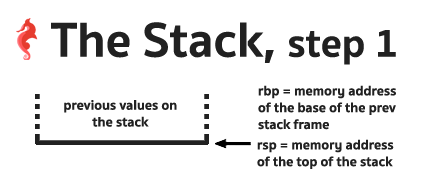• `push rbp` instruction pushes the value of the register `rbp` onto the stack. Because it “pushes” onto the stack, now the value of `rsp` is the memory address of the new top of the stack. The stack and the registers now look like this: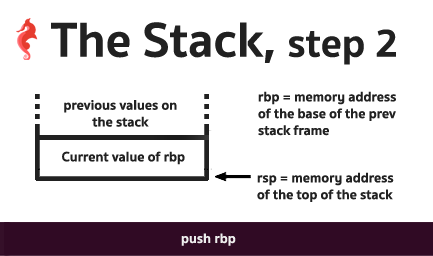• `mov rbp, rsp` copies the value of the stack pointer `rsp` to the base pointer `rbp` -> `rpb` and `rsp` now both point to the top of the stack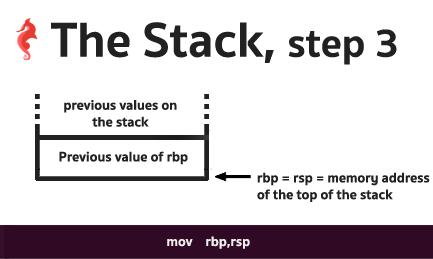• `sub rsp, 0x10` creates a space to store values of local variables. The space between `rbp` and `rsp` is this space. Note that this space is large enough to store our variable of type `integer`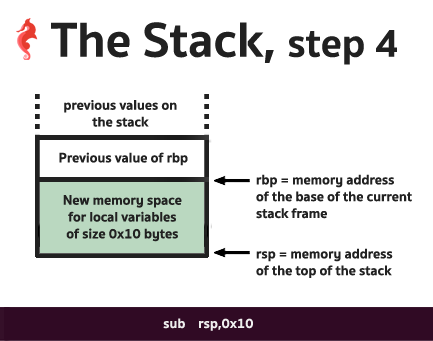We have just created a space in memory – on the stack – for our local variables. This space is called a stack frame. Every function that has local variables will use a stack frame to store those variables.

## Using local variables

The fourth line of assembly code of our `main` function is the following:

``````  400535:       c7 45 fc cc 03 00 00    mov    DWORD PTR [rbp-0x4],0x3cc
``````

`0x3cc` is actually the value `972` in hexadecimal. This line corresponds to our C-code line:

``````a = 972;
``````

`mov DWORD PTR [rbp-0x4],0x3cc` is setting the memory at address `rbp - 4` to `972`. `[rbp - 4]` IS our local variable `a`. The computer doesn’t actually know the name of the variable we use in our code, it simply refers to memory addresses on the stack.

This is the state of the stack and the registers after this operation: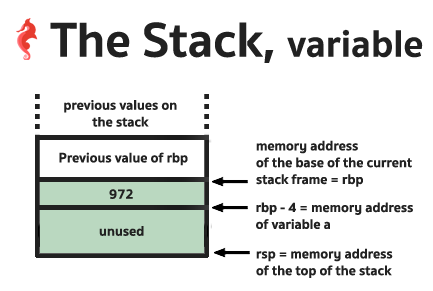## `leave`, Automatic de-allocation

If we look now at the end of the function, we will find this:

``````  400555:       c9                      leave
``````

The instruction `leave` sets `rsp` to `rbp`, and then pops the top of the stack into `rbp`.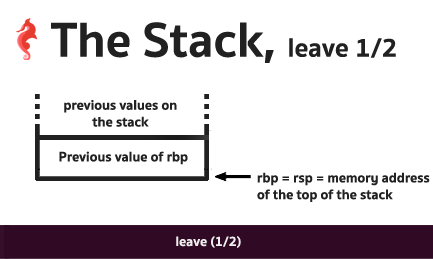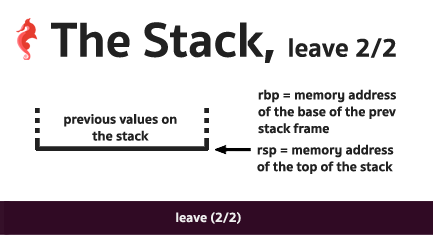Because we pushed the previous value of `rbp` onto the stack when we entered the function, `rbp` is now set to the previous value of `rbp`. This is how:

• The local variables are “de-allocated”, and
• the stack frame of the previous function is restored before we leave the current function.

The state of the stack and the registers `rbp` and `rsp` are restored to the same state as when we entered our `main` function.

## Playing with the stack

When the variables are automatically de-allocated from the stack, they are not completely “destroyed”. Their values are still in memory, and this space will potentially be used by other functions.

This is why it is important to initialize your variables when you write your code, because otherwise, they will take whatever value there is on the stack at the moment when the program is running.

Let’s consider the following C code (`1-main.c`):

``````#include <stdio.h>

void func1(void)
{
int a;
int b;
int c;

a = 98;
b = 972;
c = a + b;
printf("a = %d, b = %d, c = %d\n", a, b, c);
}

void func2(void)
{
int a;
int b;
int c;

printf("a = %d, b = %d, c = %d\n", a, b, c);
}

int main(void)
{
func1();
func2();
return (0);
}
``````

As you can see, `func2` does not set the values of its local vaiables `a`, `b` and `c`, yet if we compile and run this program it will print…

``````holberton\$ gcc 1-main.c && ./a.out
a = 98, b = 972, c = 1070
a = 98, b = 972, c = 1070
holberton\$
``````

… the same variable values of `func1`! This is because of how the stack works. The two functions declared the same amount of variables, with the same type, in the same order. Their stack frames are exactly the same. When `func1` ends, the memory where the values of its local variables reside are not cleared – only `rsp` is incremented.
As a consequence, when we call `func2` its stack frame sits at exactly the same place of the previous `func1` stack frame, and the local variables of `func2` have the same values of the local variables of `func1` when we left `func1`.

Let’s examine the assembly code to prove it:

``````holberton\$ objdump -d -j .text -M intel
``````
``````000000000040052d <func1>:
40052d:       55                      push   rbp
40052e:       48 89 e5                mov    rbp,rsp
400531:       48 83 ec 10             sub    rsp,0x10
400535:       c7 45 f4 62 00 00 00    mov    DWORD PTR [rbp-0xc],0x62
40053c:       c7 45 f8 cc 03 00 00    mov    DWORD PTR [rbp-0x8],0x3cc
400543:       8b 45 f8                mov    eax,DWORD PTR [rbp-0x8]
400546:       8b 55 f4                mov    edx,DWORD PTR [rbp-0xc]
40054b:       89 45 fc                mov    DWORD PTR [rbp-0x4],eax
40054e:       8b 4d fc                mov    ecx,DWORD PTR [rbp-0x4]
400551:       8b 55 f8                mov    edx,DWORD PTR [rbp-0x8]
400554:       8b 45 f4                mov    eax,DWORD PTR [rbp-0xc]
400557:       89 c6                   mov    esi,eax
400559:       bf 34 06 40 00          mov    edi,0x400634
40055e:       b8 00 00 00 00          mov    eax,0x0
400563:       e8 a8 fe ff ff          call   400410 <printf@plt>
400568:       c9                      leave
400569:       c3                      ret

000000000040056a <func2>:
40056a:       55                      push   rbp
40056b:       48 89 e5                mov    rbp,rsp
40056e:       48 83 ec 10             sub    rsp,0x10
400572:       8b 4d fc                mov    ecx,DWORD PTR [rbp-0x4]
400575:       8b 55 f8                mov    edx,DWORD PTR [rbp-0x8]
400578:       8b 45 f4                mov    eax,DWORD PTR [rbp-0xc]
40057b:       89 c6                   mov    esi,eax
40057d:       bf 34 06 40 00          mov    edi,0x400634
400582:       b8 00 00 00 00          mov    eax,0x0
400587:       e8 84 fe ff ff          call   400410 <printf@plt>
40058c:       c9                      leave
40058d:       c3                      ret

000000000040058e <main>:
40058e:       55                      push   rbp
40058f:       48 89 e5                mov    rbp,rsp
400592:       e8 96 ff ff ff          call   40052d <func1>
400597:       e8 ce ff ff ff          call   40056a <func2>
40059c:       b8 00 00 00 00          mov    eax,0x0
4005a1:       5d                      pop    rbp
4005a2:       c3                      ret
4005a3:       66 2e 0f 1f 84 00 00    nop    WORD PTR cs:[rax+rax*1+0x0]
4005aa:       00 00 00
4005ad:       0f 1f 00                nop    DWORD PTR [rax]
``````

As you can see, the way the stack frame is formed is always consistent. In our two functions, the size of the stack frame is the same since the local variables are the same.

``````push   rbp
mov    rbp,rsp
sub    rsp,0x10
``````

And both functions end with the `leave` statement.

The variables `a`, `b` and `c` are referenced the same way in the two functions:

• `a` lies at memory address `rbp - 0xc`
• `b` lies at memory address `rbp - 0x8`
• `c` lies at memory address `rbp - 0x4`

Note that the order of those variables on the stack is not the same as the order of those variables in our code. The compiler orders them as it wants, so you should never assume the order of your local variables in the stack.

So, this is the state of the stack and the registers `rbp` and `rsp` before we leave `func1`: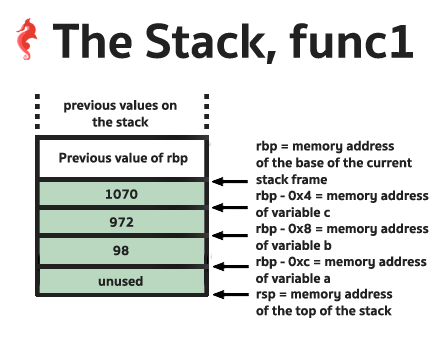When we leave the function `func1`, we hit the instruction `leave`; as previously explained, this is the state of the stack, `rbp` and `rsp` right before returning to the function `main`: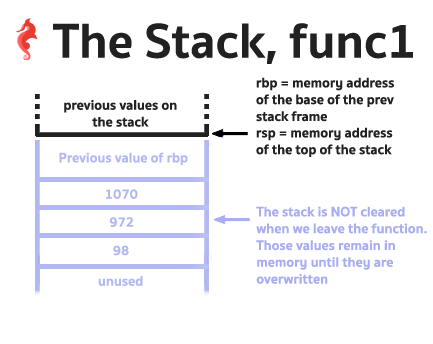So when we enter `func2`, the local variables are set to whatever sits in memory on the stack, and that is why their values are the same as the local variables of the function `func1`.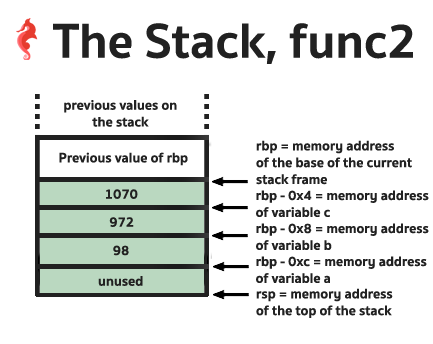## ret

You might have noticed that all our example functions end with the instruction `ret`. `ret` pops the return address from stack and jumps there. When functions are called the program uses the instruction `call` to push the return address before it jumps to the first instruction of the function called.
This is how the program is able to call a function and then return from said function the calling function to execute its next instruction.

So this means that there are more than just variables on the stack, there are also memory addresses of instructions. Let’s revisit our `1-main.c` code.

When the `main` function calls `func1`,

``````  400592:       e8 96 ff ff ff          call   40052d <func1>
``````

it pushes the memory address of the next instruction onto the stack, and then jumps to `func1`.
As a consequence, before executing any instructions in `func1`, the top of the stack contains this address, so `rsp` points to this value.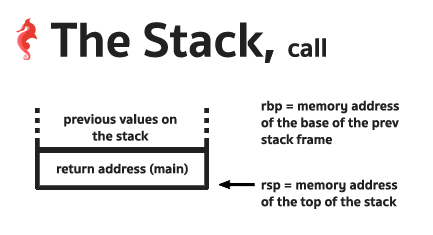After the stack frame of `func1` is formed, the stack looks like this: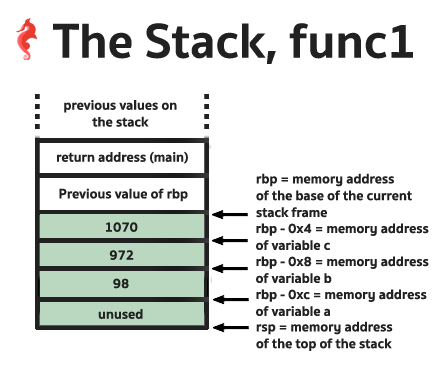## Wrapping everything up

Given what we just learned, we can directly use `rbp` to directly access all our local variables (without using the C variables!), as well as the saved `rbp` value on the stack and the return address values of our functions.

To do so in C, we can use:

``````    register long rsp asm ("rsp");
register long rbp asm ("rbp");
``````

Here is the listing of the program `2-main.c`:

``````#include <stdio.h>

void func1(void)
{
int a;
int b;
int c;
register long rsp asm ("rsp");
register long rbp asm ("rbp");

a = 98;
b = 972;
c = a + b;
printf("a = %d, b = %d, c = %d\n", a, b, c);
printf("func1, rpb = %lx\n", rbp);
printf("func1, rsp = %lx\n", rsp);
printf("func1, a = %d\n", *(int *)(((char *)rbp) - 0xc) );
printf("func1, b = %d\n", *(int *)(((char *)rbp) - 0x8) );
printf("func1, c = %d\n", *(int *)(((char *)rbp) - 0x4) );
printf("func1, previous rbp value = %lx\n", *(unsigned long int *)rbp );
printf("func1, return address value = %lx\n", *(unsigned long int *)((char *)rbp + 8) );
}

void func2(void)
{
int a;
int b;
int c;
register long rsp asm ("rsp");
register long rbp asm ("rbp");

printf("func2, a = %d, b = %d, c = %d\n", a, b, c);
printf("func2, rpb = %lx\n", rbp);
printf("func2, rsp = %lx\n", rsp);
}

int main(void)
{
register long rsp asm ("rsp");
register long rbp asm ("rbp");

printf("main, rpb = %lx\n", rbp);
printf("main, rsp = %lx\n", rsp);
func1();
func2();
return (0);
}
``````

### Getting the values of the variablesFrom our previous discoveries, we know that our variables are referenced via `rbp` – 0xX:

• `a` is at `rbp - 0xc`
• `b` is at `rbp - 0x8`
• `c` is at `rbp - 0x4`

So in order to get the values of those variables, we need to dereference `rbp`. For the variable `a`:

• cast our variable `rbp` to a `char *`: `(char *)rbp`
• subtract the correct amount of bytes to get the address of where the variable is in memory: `(char *)rbp) - 0xc`
• cast it again to a pointer pointing to an `int` since `a` is of type `int`: `(int *)(((char *)rbp) - 0xc)`
• and dereference it to get the value sitting at this address: `*(int *)(((char *)rbp) - 0xc)`

### The saved `rbp` valueLooking at the above diagram, the current `rbp` directly points to the saved `rbp`, so we simply have to cast our variable `rbp` to a pointer to an `unsigned long int` and dereference it: `*(unsigned long int *)rbp`.The return address value is right before the saved previous `rbp` on the stack. `rbp` is 8 bytes long, so we simply need to add 8 to the current value of `rbp` to get the address where this return value is on the stack. This is how we do it:

• cast our variable `rbp` to a `char *`: `(char *)rbp`
• add 8 to this value: ((char *)rbp + 8)
• cast it to point to an `unsigned long int`: `(unsigned long int *)((char *)rbp + 8)`
• dereference it to get the value at this address: `*(unsigned long int *)((char *)rbp + 8)`

### The output of our program

``````holberton\$ gcc 2-main.c && ./a.out
main, rpb = 7ffc78e71b70
main, rsp = 7ffc78e71b70
a = 98, b = 972, c = 1070
func1, rpb = 7ffc78e71b60
func1, rsp = 7ffc78e71b50
func1, a = 98
func1, b = 972
func1, c = 1070
func1, previous rbp value = 7ffc78e71b70
func1, return address value = 400697
func2, a = 98, b = 972, c = 1070
func2, rpb = 7ffc78e71b60
func2, rsp = 7ffc78e71b50
holberton\$
``````

We can see that:

• from `func1` we can access all our variables correctly via `rbp`
• from `func1` we can get the `rbp` of the function `main`
• we confirm that `func1` and `func2` do have the same `rbp` and `rsp` values
• the difference between `rsp` and `rbp` is 0x10, as seen in the assembly code (`sub rsp,0x10`)
• in the `main` function, `rsp` == `rbp` because there are no local variables

The return address from `func1` is `0x400697`. Let’s double check this assumption by disassembling the program. If we are correct, this should be the address of the instruction right after the call of `func1` in the `main` function.

``````holberton\$ objdump -d -j .text -M intel | less
``````
``````0000000000400664 <main>:
400664:       55                      push   rbp
400665:       48 89 e5                mov    rbp,rsp
400668:       48 89 e8                mov    rax,rbp
40066b:       48 89 c6                mov    rsi,rax
40066e:       bf 3b 08 40 00          mov    edi,0x40083b
400673:       b8 00 00 00 00          mov    eax,0x0
400678:       e8 93 fd ff ff          call   400410 <printf@plt>
40067d:       48 89 e0                mov    rax,rsp
400680:       48 89 c6                mov    rsi,rax
400683:       bf 4c 08 40 00          mov    edi,0x40084c
400688:       b8 00 00 00 00          mov    eax,0x0
40068d:       e8 7e fd ff ff          call   400410 <printf@plt>
400692:       e8 96 fe ff ff          call   40052d <func1>
400697:       e8 7a ff ff ff          call   400616 <func2>
40069c:       b8 00 00 00 00          mov    eax,0x0
4006a1:       5d                      pop    rbp
4006a2:       c3                      ret
4006a3:       66 2e 0f 1f 84 00 00    nop    WORD PTR cs:[rax+rax*1+0x0]
4006aa:       00 00 00
4006ad:       0f 1f 00                nop    DWORD PTR [rax]
``````

And yes! \o/

## Hack the stack!

Now that we know where to find the return address on the stack, what if we were to modify this value? Could we alter the flow of a program and make `func1` return to somewhere else? Let’s add a new function, called `bye` to our program (`3-main.c`):

``````#include <stdio.h>
#include <stdlib.h>

void bye(void)
{
printf("[x] I am in the function bye!\n");
exit(98);
}

void func1(void)
{
int a;
int b;
int c;
register long rsp asm ("rsp");
register long rbp asm ("rbp");

a = 98;
b = 972;
c = a + b;
printf("a = %d, b = %d, c = %d\n", a, b, c);
printf("func1, rpb = %lx\n", rbp);
printf("func1, rsp = %lx\n", rsp);
printf("func1, a = %d\n", *(int *)(((char *)rbp) - 0xc) );
printf("func1, b = %d\n", *(int *)(((char *)rbp) - 0x8) );
printf("func1, c = %d\n", *(int *)(((char *)rbp) - 0x4) );
printf("func1, previous rbp value = %lx\n", *(unsigned long int *)rbp );
printf("func1, return address value = %lx\n", *(unsigned long int *)((char *)rbp + 8) );
}

void func2(void)
{
int a;
int b;
int c;
register long rsp asm ("rsp");
register long rbp asm ("rbp");

printf("func2, a = %d, b = %d, c = %d\n", a, b, c);
printf("func2, rpb = %lx\n", rbp);
printf("func2, rsp = %lx\n", rsp);
}

int main(void)
{
register long rsp asm ("rsp");
register long rbp asm ("rbp");

printf("main, rpb = %lx\n", rbp);
printf("main, rsp = %lx\n", rsp);
func1();
func2();
return (0);
}
``````

Let’s see at which address the code of this function starts:

``````holberton\$ gcc 3-main.c && objdump -d -j .text -M intel | less
``````
``````00000000004005bd <bye>:
4005bd:       55                      push   rbp
4005be:       48 89 e5                mov    rbp,rsp
4005c1:       bf d8 07 40 00          mov    edi,0x4007d8
4005c6:       e8 b5 fe ff ff          call   400480 <puts@plt>
4005cb:       bf 62 00 00 00          mov    edi,0x62
4005d0:       e8 eb fe ff ff          call   4004c0 <exit@plt>
``````

Now let’s replace the return address on the stack from the `func1` function with the address of the beginning of the function `bye`, `4005bd` (`4-main.c`):

``````#include <stdio.h>
#include <stdlib.h>

void bye(void)
{
printf("[x] I am in the function bye!\n");
exit(98);
}

void func1(void)
{
int a;
int b;
int c;
register long rsp asm ("rsp");
register long rbp asm ("rbp");

a = 98;
b = 972;
c = a + b;
printf("a = %d, b = %d, c = %d\n", a, b, c);
printf("func1, rpb = %lx\n", rbp);
printf("func1, rsp = %lx\n", rsp);
printf("func1, a = %d\n", *(int *)(((char *)rbp) - 0xc) );
printf("func1, b = %d\n", *(int *)(((char *)rbp) - 0x8) );
printf("func1, c = %d\n", *(int *)(((char *)rbp) - 0x4) );
printf("func1, previous rbp value = %lx\n", *(unsigned long int *)rbp );
printf("func1, return address value = %lx\n", *(unsigned long int *)((char *)rbp + 8) );
/* hack the stack! */
*(unsigned long int *)((char *)rbp + 8) = 0x4005bd;
}

void func2(void)
{
int a;
int b;
int c;
register long rsp asm ("rsp");
register long rbp asm ("rbp");

printf("func2, a = %d, b = %d, c = %d\n", a, b, c);
printf("func2, rpb = %lx\n", rbp);
printf("func2, rsp = %lx\n", rsp);
}

int main(void)
{
register long rsp asm ("rsp");
register long rbp asm ("rbp");

printf("main, rpb = %lx\n", rbp);
printf("main, rsp = %lx\n", rsp);
func1();
func2();
return (0);
}
``````
``````holberton\$ gcc 4-main.c && ./a.out
main, rpb = 7fff62ef1b60
main, rsp = 7fff62ef1b60
a = 98, b = 972, c = 1070
func1, rpb = 7fff62ef1b50
func1, rsp = 7fff62ef1b40
func1, a = 98
func1, b = 972
func1, c = 1070
func1, previous rbp value = 7fff62ef1b60
func1, return address value = 40074d
[x] I am in the function bye!
holberton\$ echo \$?
98
holberton\$
``````

We have called the function `bye`, without calling it!## Outro

I hope that you enjoyed this and learned a couple of things about the stack. As usual, this will be continued! Let me know if you have anything you would like me to cover in the next chapter.

### Questions? Feedback?

If you have questions or feedback don’t hesitate to ping us on Twitter at @holbertonschool or @julienbarbier42.
Haters, please send your comments to `/dev/null`.

Happy Hacking!

This repo contains the source code (`X-main.c` files) for programs created in this tutorial.
Many thanks to Naomi for proof-reading!